﻿

### On the edge metric dimension and Wiener index of the blow up of graphs

#### Abstract

Let$G=(V,E)$ be a connected graph. The distance between an edge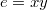$e=xy$ and a vertex$v$ is defined as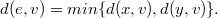$\T{d}(e,v)=\T{min}\{\T{d}(x,v),\T{d}(y,v)\}.$ A nonempty set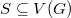$S \subseteq V(G)$ is an edge metric generator for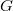$G$ if for any two distinct edges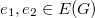$e_1,e_2 \in E(G)$, there exists a vertex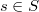$s \in S$ such that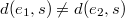$\T{d}(e_1,s) \neq \T{d}(e_2,s)$. An edge metric generating set with the smallest number of elements is called an edge metric basis of$G$, and the number of elements in an edge metric basis is called the edge metric dimension of$G$ and it is denoted by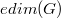$\T{edim}(G)$. In this paper, we study the edge metric dimension of a blow up of a graph$G$, and also we study the edge metric dimension of the zero divisor graph of the ring of integers modulo$n$. Moreover, the Wiener index and the hyper-Wiener index of the blow up of certain graphs are computed.

DOI Code: 10.1285/i15900932v40n2p99

Keywords: Edge metric dimension; Wiener index; Hyper-Wiener index; Blow up of a graph; Zero divisor graph

Full Text: PDF